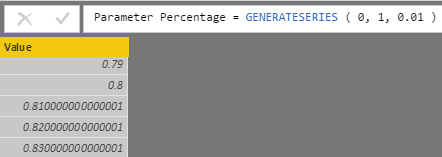## The GENERATESERIES function

The August 2017 update of Power BI introduced a new feature called the What if parameter, which allows the use of a slicer to push a parameter in a DAX measure. Internally, this feature simply creates a calculated table using a DAX expression generating one row for each value that should be available in the slicer.

The DAX expression uses a new function called GENERATESERIES. GENERATESERIES  is one of the few functions in DAX that generates new data – most of the DAX functions only filter existing data. The syntax is the following:

```GENERATESERIES ( <StartValue>, <EndValue> [, <IncrementValue>] )
```

The result is a table with a single column called Value. The first two arguments define the first and last value of the table. The third optional argument defines the increment applied when generating the numbers: it is 1 by default, but you can apply any other positive value including decimal numbers. The data type of the result is an integer if the values are all integers, or a decimal number if one or more values require that level of precision. Here are a few examples of GENERATESERIES.

Generating all the integer numbers between 0 and 100:

```= GENERATESERIES ( 1, 100 )
```

Generating all the even numbers between -10 and 10:

```= GENERATESERIES ( -10, 10, 2 )
```

Generating all the numbers between 0% and 100%:

```= GENERATESERIES ( 0, 1, 0.01 )
```

The last function generates numbers using a floating-point value. This can result in numbers that are not accurate for your needs. For example, instead of generating 0.81, you would see the number 0.810000000000001 in the result as shown below:Usually this is not an issue if you just display the number formatted using a percentage with two decimal points. In case you need more accuracy you can just convert the number in a fixed decimal number, using the CURRENCY function as in the following example:

```= SELECTCOLUMNS (
GENERATESERIES ( 0, 1, 0.01 ),
"Value", CURRENCY ( [Value] )
)
```

The GENERATESERIES function is available in Power BI, Azure Analysis Services, and Analysis Services 2017.

## Alternative to GENERATESERIES function

If you create a live connection between Power BI and Analysis Services 2016, or if you use Power Pivot for Excel, you cannot use the GENERATESERIES function. However, you can easily work around the absence of GENERATESERIES by using the CALENDAR function, which is available in Power Pivot for Excel 2016 and Analysis Services 2016.

The CALENDAR function generates all the dates between two dates. Because a date is a floating-point number, you can easily manipulate this function as needed to get the desired numerical result. The only issue is that you cannot specify a step parameter, so the number generated should be modified using   the SELECTCOLUMNS function. Here are the same previous examples written using CALENDAR instead of GENERATESERIES.

Generating all the integer numbers between 0 and 100:

```= SELECTCOLUMNS ( CALENDAR ( 0, 100 ), "Value", INT ( [Date] ) )
```

Generating all the even numbers between -10 and 10:

```= SELECTCOLUMNS ( CALENDAR ( -5, 5 ), "Value", INT ( [Date] * 2 ) )
```

Generating all the numbers between 0% and 100%:

```= SELECTCOLUMNS ( CALENDAR ( 0, 100 ), "Value", CURRENCY ( [Date] / 100 ) )
```

Using the expressions above, you can implement the Parameter Table pattern using a calculated table in Analysis Services 2016. You cannot create a calculated table in Power Pivot for Excel, so in that case this technique would only be useful to generate more complex DAX expressions requiring a table with a list of numbers.

In order to get the selected value in Analysis Services 2016 – where you do not have the SELECTEDVALUE function used by the What If Parameters in Power BI – you can use the following DAX syntax:

```Parameter Value =
IF (
HASONEVALUE ( Parameter[Parameter] ),
VALUES ( Parameter[Parameter] )
)
```

The Power BI file that you can download contains all the examples described in this article, including those based on the syntax available in Analysis Services 2016.

GENERATESERIESReturns a table with one column, populated with sequential values from start to end.

`GENERATESERIES ( <StartValue>, <EndValue> [, <IncrementValue>] )`

CURRENCYReturns the value as a currency data type.

`CURRENCY ( <Value> )`

CALENDARReturns a table with one column of all dates between StartDate and EndDate.

`CALENDAR ( <StartDate>, <EndDate> )`

SELECTCOLUMNSReturns a table with selected columns from the table and new columns specified by the DAX expressions.

`SELECTCOLUMNS ( <Table> [[, <Name>], <Expression> [[, <Name>], <Expression> [, … ] ] ] )`

SELECTEDVALUEReturns the value when there’s only one value in the specified column, otherwise returns the alternate result.

`SELECTEDVALUE ( <ColumnName> [, <AlternateResult>] )`